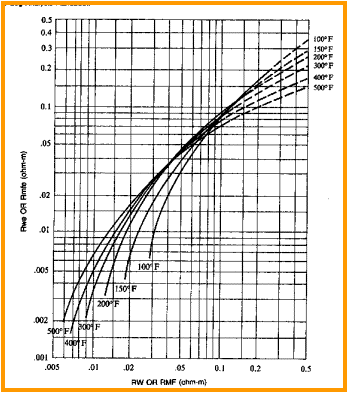Water Resistivity FROM SP LOG
Calculation from knowledge of the SP value in a clean zone has been a traditional method  for finding RW. It works best in clean water bearing zones, but the Rwa or R0 method would be better in this case. Shale content and hydrocarbon content reduce the SP value and cause RW to be too high, giving pessimistic saturation results. However, if you have no other helpful RW data, start here and use good judgement.RW from SP MODEL
This is the official 1980 vintage algorithm courtesy of the Bateman and Konen programmable calculator paper. It honours the chartbook solution quite closely:
1: FT = SUFT + (BHT - SUFT) / BHTDEP * DEPTH
2: IF LOGUNITS\$ = "METRIC"
3: THEN FT1 = 9 / 5 * FT + 32
4: OTHERWISE FT1 = FT
5: KSP = 60 + 0.122 * FT1
6: RSP = 10 ^ (-SSP / KSP)
7: IF RMF@FT > 0.1
8: THEN RMFE = 0.85 * RMF@FT
9: IF RMF@FT <= 0.1
10: THEN RMFE = (146 * RMF@FT - 5) / (337 * RMF@FT + 77)
11: RWE = RMFE / RSP
12: IF RWE > 0.12
13: THEN RW@FT = - (0.58 - 10 ^ (0.69 * RWE - 0.24))
14: IF RWE <= 0.12
15: THEN RW@FT = (77 * RWE + 5) / (146 - 337 * RWE)

Where:
BHT = bottom hole temperature (degrees Fahrenheit or Celsius)
BHTDEP = depth at which BHT was measured (feet or meters)
DEPTH = mid-point depth of reservoir (feet or meters)
FT1 = formation temperature (degrees Fahrenheit)
KSP = temperature factor (degrees Fahrenheit)
RSP = RWE / RMFE (fractional)
RMFE = equivalent mud filtrate resistivity (ohm-m)
RMF@FT = mud filtrate resistivity at formation temperature (ohm-m)
RWE = equivalent water resistivity (ohm-m)
RW@FT = water resistivity at formation temperatures (ohm-m)
SSP = static SP reading in clean zone (ohm-m)
SUFT = surface temperature (degrees Fahrenheit or Celsius)COMMENTS:
This algorithm should only be used IF the SP log has sufficient character, the zone of interest is a clean water bearing sandstone, and the result is reasonable for the area. Use caution since many SP logs are not calibrated, and RMF or RW can be measured carelessly.

Solution of these formulae can be done on the two charts below. An Excel macro for RW from SP, published by SPWLA, is located HERE.Nomographs for RW from SPNUMERICAL EXAMPLE:
1. Data for Sand C:
SUFT = 25 degrees C
BHT = 65 degrees C
BHTDEP = 2225 m
DEPTH = 1000 m
RMF = 0.75 @ 25 degrees C
SP = -90 mv

FT = 25 + (65 - 25) / 2225 * 1000 = 43 degrees C
FT1 = 9 / 5 * 43 + 32 = 109 degrees F
RMF@FT = 0.75 * (25 + 21.5) / (43 + 21.5) = 0.54
KSP = 60 + 0.122 * 109 = 73.3
RSP = 10 ^ (90 / 73.3) = 16.9
RMFE = 0.85 * 0.54 = 0.46
RWE = 0.46 / 16.9 = 0.027
RW@FT = (77 * 0.027 + 5) / (146 - 337 * 0.027) = 0.051

Page Views ---- Since 01 Jan 2015